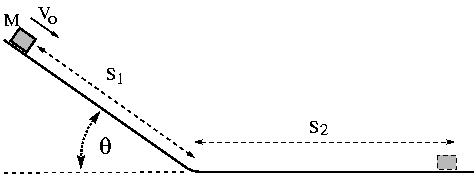Kinetic friction on incline/flat surface system

Homework Statement

http://nplq1.phyast.pitt.edu/res/msu/physicslib/msuphysicslib/13_EnergyConservation/graphics/prob27a_MechEnWFriction.gif

When mass M is at the position shown, it is sliding down the inclined part of a slide at a speed of 2.07 m/s. The mass stops a distance S2 = 1.9 m along the level part of the slide. The distance S1 = 1.18 m and the angle q = 32.50°. Calculate the coefficient of kinetic friction for the mass on the surface.

Homework Equations

F=ma
$$v^2=v_0^2+2ax$$
$$\mu_k=\frac{f}{F_n}=\frac{mgsin(\theta)-ma}{mgcos(\theta)}$$

The Attempt at a Solution

OK, I've been having trouble with this problem for a while now. Basicaly using the equations above I've tried to solve for a and plug that into the last formula there to get the coefficient of friction. However, this is proving to be very difficult for me, as I can't seem to combine the motion on the incline and the motion on the flat surface to find out what a on the incline is. I'm assuming that the accelerations are different for the two sections, and there is no indication that the coefficient is the same on the flat surface. I'm only interested in the friction on the incline, I believe.

Anyway, if anyone could offer a pointer I'd greatly appreciate it. I've been working on this a while and I can't seem to tell if I'm on the right track. Thanks!

Last edited by a moderator:

wheres the picture?

I already posted the pic, maybe you can't see it for some reason. Here's a link:

EDIT: nevermind

Last edited:
It seems I can't post the picture because it's from a site that has to be logged into. Basically, to the left is an incline on which the block starts. The length of the incline is the s1 listed. Then at the bottom of the incline the path levels off and the length of the level part is s2. The block starts out on the top of the incline with the initial speed...this is the "position shown" referred to in the question.

ANY help would be super-appreciated.

No one has any clue?

bump